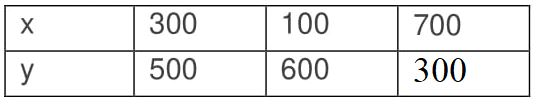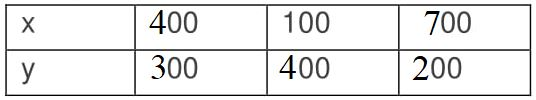Guru

# The coach of a cricket team buys 3 bats and 6 balls for Rs.3900. Later, she buys another bat and 3 more balls of the same kind for Rs.1300. Represent this situation algebraically and geometrically. Q.2

• 0

This is the best question of the chapter of pair of linear equations in two variables but i am not getting the best answer of this question please help for solving this question The coach of a cricket team buys 3 bats and 6 balls for Rs.3900. Later, she buys another bat and 3 more balls of the same kind for Rs.1300. Represent this situation algebraically and geometrically.

Share

1. Let us assume that the cost of a bat be ‘Rs x’

And,the cost of a ball be ‘Rs y’

According to the question, the algebraic representation is

3x+6y = 3900

And x+3y = 1300

For, 3x+6y = 3900

Or x = (3900-6y)/3

The solution table isFor, x+3y = 1300

Or x = 1300-3y

The solution table is• 0